# Fourier Transform: Data approximation

From the work of Fourier(1768-1830) we know that every integrable function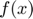defined in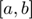can be approximate by a sum of trigonometric functions: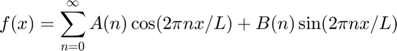.

Here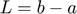and because Fourier transform is specilly appropiate for periodic functions we can make a change of variables in the x variables a get the expressions for the Fourier coefficients as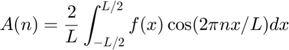and analogously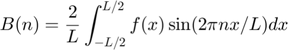Compact representation:

If we define Phases as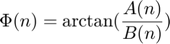and amplitudes as, Fourier transform can be also written as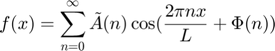.

## A Simple Example:

Consider one funcion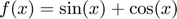defined on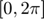and we evaluateon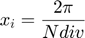where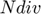is the number of subdivisions.

clear all;
close all;
Ndiv=8;
h=2*pi/Ndiv;
x=0:h:2*pi-h; %don't take last point (it is supposed to be the same as 0)
f=sin(x)+cos(x);

N=length(f);


## Compute Discrete Fourier Transform

For N values we consider the points in the interval [-N/2, N/2] (this is more stable from a numerical point of view) and we approximate the integral by the sumatory (trapezoidal's rule).

By deffault we compute as many Fourier coefficients than number of points.

for k=0:N-1
sumA=0;
sumB=0;
for j=0:N-1
sumA=sumA+f(j+1)*cos(2*pi*j*(k-N/2)/N);
sumB=sumB+f(j+1)*sin(2*pi*j*(k-N/2)/N);
end
A(k+1)=sumA/N;
B(k+1)=sumB/N;
end


## Compute Inverse Fourier Transform

Once we have the Fourier coefficients we can use the Fourier series to approximate the true value f at each point.

We increase the order approximation to visualize convergence of the sumatory. Notice that only 3 coefficients are enought for this particular function

for order=1:N-1
plot(x,f,'o-'); %plot the original function
hold on;
for k=0:N-1
sum=0;
for j=0:order
sum=sum+A(j+1)*cos(2*pi*k*(j-N/2)/N)+B(j+1)*sin(2*pi*k*(j-N/2)/N);
end
faprox(k+1)=sum;
end
plot(x,faprox,'*-'); %plot the approximated points
Error(order)=max(abs(f-faprox));
end
hold off;
disp('Error at each order approximation');
Error'

Error at each order approximation

ans =

1.4142
1.4142
0.7071
0.7071
0.0000
0.0000
0.0000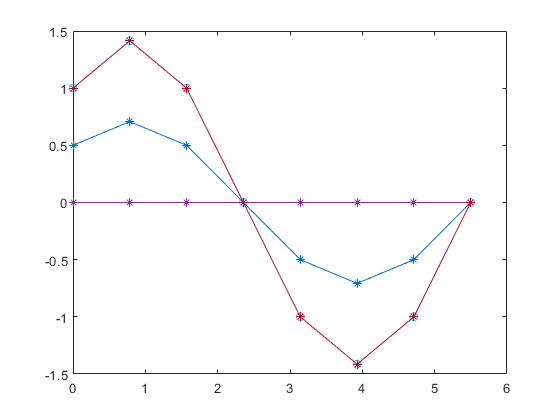## Frequencies and Magnitudes

We can represent the frequences space and the associated magnitudes from the previous formulas

for i=1:N
Atilde(i)=sqrt(A(i)^2+B(i)^2);
Phi(i)=atan(B(i)/A(i));
end
figure()
subplot(2,1,1)
stem(-N/2:N/2-1,Atilde)
xlabel('frequency');ylabel('amplitude')
subplot(2,1,2)
stem(-N/2:N/2-1,Phi)
xlabel('frequency');ylabel('phase angle')## General Data Approach: Function through a data set.

Consider now that you have a set of measure data and you want to compute a function passing through these data. The Fourier approximation allow us to compute this function.

We will define (at the bottom of this document) the two functions:

• Discrete Fourier Transform: function fcoeff=DiscFourierTrans(f,N)
• Inverse Fourier Transform: function faprox=invFourierTrans(fcoeff,N,order)
%Consider a set of data values
f=[10.7083
9.7003
8.9780
8.4531
8.1653
8.2633
8.8795
9.9850
11.3289
12.5172
13.2012
13.2720
12.9435
12.6647
12.8940
13.8516
15.3819
17.0033
18.1227
18.3039
17.4531
15.8322
13.9056
12.1154];
x=0:length(f)-1; % here x is just the enumeration of the data values.
N=length(x);
fcoeff=DiscFourierTrans(f,N);
order=15; %try order = 13, 14 to see the different approximation
faprox=invFourierTrans(fcoeff,N,order);
figure()
plot(x,f,'o-'); %plot the approximated points
hold on;
plot(x,faprox,'*-'); %plot the approximated points## General Data Using Fast Fourier Transform from Matlab

We solve the same previous example but using now Matlab function Fast Fourier Transform (fft)

## Fourier transform analysis

P1 = fft(f)/N; %fast Fourier transform
P2=fftshift(P1);
% You can recover the real coefficients
fcoeff=[real(P2),-imag(P2)];
order=15;
faprox=invFourierTrans(fcoeff,N,order); %using our function
% or you can use the compact Fourier formula
amp=abs(P2); % amplitude for complex variables
phi = angle(P2); % phase angle for complex variables
for k=0:N-1
sum=0;
for j=0:order
sum=sum+amp(j+1)*cos(2*pi*k*(j-N/2)/N+phi(j+1));
end
faproxCompact(k+1)=sum;
end

figure()
title('Now using fft')
plot(x,f,'s-'); %plot the approximated points
hold on;
plot(x,faprox,'d-'); %plot the approximated points
plot(x,faproxCompact,'*-'); %plot the approximated points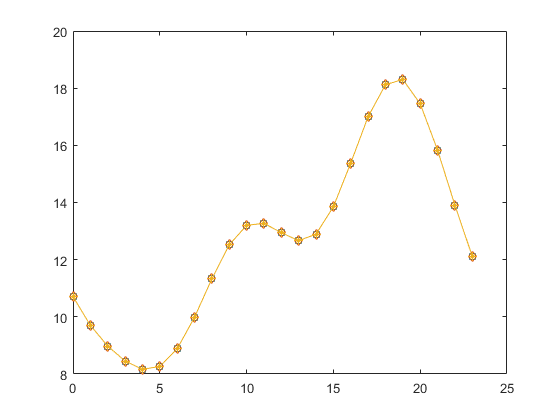## Numerical trigonometric interpolation in a new point

Once we have the Fourier approximation of a set of data, we can use it to approximate the corresponding value for an unknown point not belonging to the data set.

We can use different order approximation and see how the new value behaves. Below we show for the point 10.5 (not in the original table) how the approximations of different Fourier order fits the original interpolated curve. For the maximum order (15) the interpolated value is on the curve.

point=10.5;
order=[12,13,15];
for i=1:3
sum=0;
for j=0:order(i)
sum=sum+amp(j+1)*cos(2*pi*point*(j-N/2)/N+phi(j+1));
end
fvalue(i)=sum;
end
xx=point*ones(3,1); % just to plot
plot(xx,fvalue','sm'); %plot the approximated points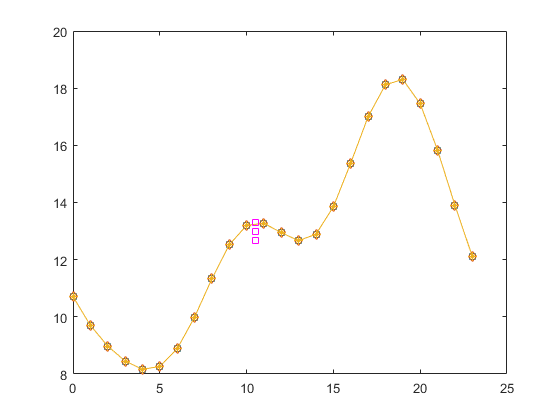## Discrete Fourier Transform Function

function fcoeff=DiscFourierTrans(f,N)
for k=0:N-1
sumA=0;
sumB=0;
for j=0:N-1
sumA=sumA+f(j+1)*cos(2*pi*j*(k-N/2)/N);
sumB=sumB+f(j+1)*sin(2*pi*j*(k-N/2)/N);
end
A(k+1)=sumA/N;
B(k+1)=sumB/N;
end
fcoeff=[A;B]';
end


## Inverse Fourier Transform Function

function faprox=invFourierTrans(fcoeff,N,order)
if (order >=N), order=N-1; end;
A=fcoeff(:,1);
B=fcoeff(:,2);
for k=0:N-1
sum=0;
for j=0:order
sum=sum+A(j+1)*cos(2*pi*k*(j-N/2)/N)+B(j+1)*sin(2*pi*k*(j-N/2)/N);
end
faprox(k+1)=sum;
end
end


## (c) Numerical Factory 2019

(see this video for more intuition https://www.youtube.com/watch?reload=9&v=spUNpyF58BY )